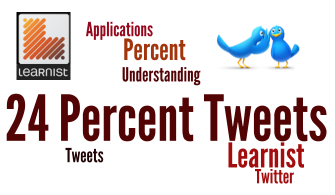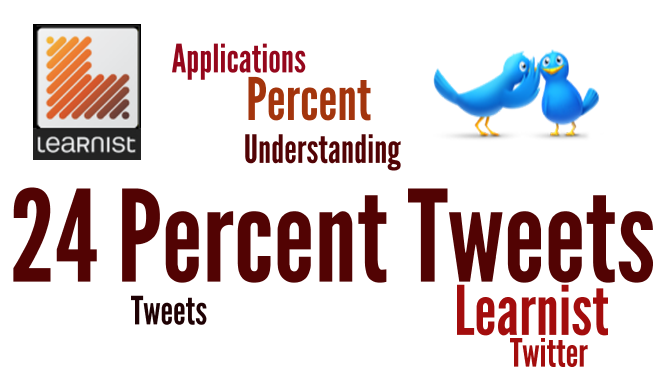# 24 Percent Tweets

Twenty 24 important things to know about percent.  Each is tweetable.  This is 24 short statements (about Percent ;-) that can be stated in 140 characters or less.No. Tweet
Explantion & Examples

SlideShare

Practice Card Deck

Quizlet

More Practice

Quizlet

1

50% is one-half, 100% is all of it (1), and 150% is one and a half2
To convert between decimals and percents move the decimal point (exactly!) 2 places3
Take half to get 50%; Take half again to get 25% (1/4); Add them to get 75% (3/4)4
To get 10% of a number move the decimal point to the left 1 place or drop 1 zero5
2 find A% of B: U can change the percent to a fraction. Find B divided by the bottom. Multiply by the top.6
2 find A% of B: U can multiply the numbers & put the decimal point in the only logical place7
2 convert a fraction 2 a percent: divide, then move the decimal place 2 places to the right, & put on %8
2 convert a percent 2 a fraction: Make it a decimal (move 2 places left & remove %) & read it correctly [simplify]9
20% is 1/5; to find 20% of a number divide by 5 (tip/time-saver: or divide by 10 and double)10

To solve _% of A is B write an equation changing _ to x; of to *; is to =; do alg. step; write x as a %.11
1/3 is 33 1/3 %; To find 33 1/3 % divide by 3. 66 2/3 % is two of them.12
A% of _ is B.  Write the equation using: ‘of’ is *; ‘_’ is x; ‘is’ is =. Then solve.13
1/8 is 12.5% - To find 12.5% divide by 8. 1/8 is 12.5% because half of 1/4 is 1/8 and half of 25% is 12.5%14
To find A% of B: U can change the percent to a fraction (or mixed number); divide the bottom into B; and multiply by the top15
1/6 is 16 2/3 % - 1/6 is 16 2/3 % because half of 1/3 is 1/6 and half of 33 1/3 % is 16 2/3 %16

Percent change = (amount of change)/(original amount) and write it as a percent.  (Day 1 of 4 on percent change)17
1/9 is about 11% because 1/3 of 1/3 is 1/9 and 1/3 of 33 1/3 % is about 11%; 7/9 is about 77%.18
If given percent change and original, the percent of the original is the amount of change. (Day 2 of 4 on percent change)19
To find 14% of a number divide by 7; 1/7 is about 14% because 7*14 = 98 (almost 100)20
B careful w percents less than 1%. Handle ‘em the same way (move exactly 2 places).21

% Remaining Method: If increasing, the % remaining is >100%. A decrease: less than 100% remains. Use orig’l*percent remain’g = new. (Day 3 of 4 on percent change)22
1/20 is 5% because Half of 10% is 5% and Half of 1/10 is 1/20 and 5*20=100.23
If given % change & amt. of change: Use Percent change=(amt of change)/(x); Do 2 alg steps 2 find x (orig’l)  (Day 4 of 4 on percent change)24
More applications – For mark-ups and mark-downs, % remain’g wks well. Also U *can* have percent change on percent data.(Note: the Learnist site is not longer working.)

## Hashtags

Materials associated with the first tweet (the first concept) will be be designated with #P1. #P2 is associated with the second tweet - the second important concept, and so on. This continues until #P24, which is associated with the 24th important percent concept (the 24th tweet).

## Background

This is a curriculum (teaching/learning materials) made up of 24 short lessons on percent. The purpose is to teach concepts and skills about percent and the use of percents in the real world. The concepts and skills are presented in short Slideshare presentations, which include explanations and examples. Students are provided with practice questions using Quizlet decks. Optional worksheets are provided below.

Understanding of percents, fractions, and decimals is both challenging for students and essential for success in mathematics and for understanding how numbers are used in our world.  I think 'the rubber really hits the road' when it comes to percent. I say this for two reasons: (1) Percent involves fractions and decimals. To effectively use percents one needs to flexibly leverage the interplay of percents, fractions, and decimals. (2) Percents are a primary tool used in our society to report on changes in our world, from ESPN reporting basketball statistics, to the news media reporting on polls, our society, and economics.

This 24 Percent Tweets project focuses on conceptual understanding and number sense, using procedural methods as tools. The majority of the questions can be done mentally (without a calculator). On the other hand, it is appropriate to use a calculator on some of the questions.

Teachers and students wishing to use worksheets, can find those below.

Note on percents greater than 100%: It is important to understand percents which are greater than 100% (for example, 125% = 1.25 = 1¼). (These are often overlooked or de-emphasized, and therefore, misunderstood.) Percents greater than 100% are included frequently in this 24 Percent Tweets curriculum, starting with #P1.

## Worksheets created from the Quizlet decks

Following each Slideshare presentation is a Quizlet deck (online flashcards) on the concept presented, with usually about 8 questions (and answers are on the back of the card). Then a 4 question/card Quizlet deck reviewing the previous concept.

The 8 questions on each new concept and the 4 review questions have been put onto a half-page worksheet. There are 24 half-page worksheets (each with about 12 questions).

#P1 to #P6
#P7 to #P12
#P13 to #P18
#P19 to #P24

## Audiences

This curriculum is for anyone who needs (or wants :-) to learn about percents and their uses in the real-world. This might include:

• 7th graders - under the CCSSM, 7th graders are expected to have a thorough understanding of percent and its applications.
• Algebra students who will be studying exponential functions. To grasp exponential functions one needs to understand percent change.
• Business students, who use percent on a regular basis. Fluency with percents is useful for business decision-making.

## Uses - a variety of ways

Slideshare presentations can be used for worked examples and demonstrations.

Quizlet decks can be used for in-class exercises or out-of-class practice.

I use these learning materials at the beginning of most class periods in my 100-level algebra course. (I teach at Western Illinois University.) I show the Slideshare presentation, which takes about 5 minutes (or less). Students then work (for about 5 minutes) on the (written) worksheets. (I walk around the classroom answering questions and helping students.) We usually go over the answers (takes about 2 minutes). One concept (one "Percent Tweet") each day.

The 24 concepts are presented in a logical order. However, the Slideshare presentations are independent of one another.

## Overview of What is Included

Click here for a List of all 24 Tweets (concepts/things to know). Included in the 24 concepts (but not in this order) you will find:

• Fraction-Decimal-Percent conversion.
• How to solve each of the following: A% of B is ___;  A% of ___ is B;   __% of A is B.
• Percent equivalents for every unit fraction from 1/2 = 50%, ⅓ = 33⅓%, ¼ = 25%, ... , 1/9 ≈ 11%.
• Percent change problems - including all 6 percent change problem types. A graphic organizer is presented to assist students doing percent change problems. The percent remaining method is presented and utilized.
• Work with percents greater than 100% (250%, for example) and percents less than 1% (.08%, for example).
• Applications are included in every Slideshare presentation, every Quizlet deck, and on every worksheet.
• Mental math strategies. For example, to find 75% of 800 one can use ¾. In particular, divide by 4 first and then multiply by 3. On the other hand, to find 60% of 800, one can multiply 6×8 and put on the only logical number of zeros (1 zero): 60% of 800 = 480.

##Twitter

If you want to follow me on Twitter, click @DrOlsen314.

## Percent Change Tweets

Four of the 24 'tweets' are on percent change (#16, #18, #21, #23).

Back to Jim Olsen's homepage

24 Percent Tweets Information Page
Page URL: http://www.wiu.edu/users/mfjro1/wiu/BootCamp/24-percent-tweets.html (shortcut: http://bit.ly/ZXLw0I)
©James R. Olsen, Western Illinois University. E-mail: jr-olsen 'at' wiu.edu
updated February 3, 2017